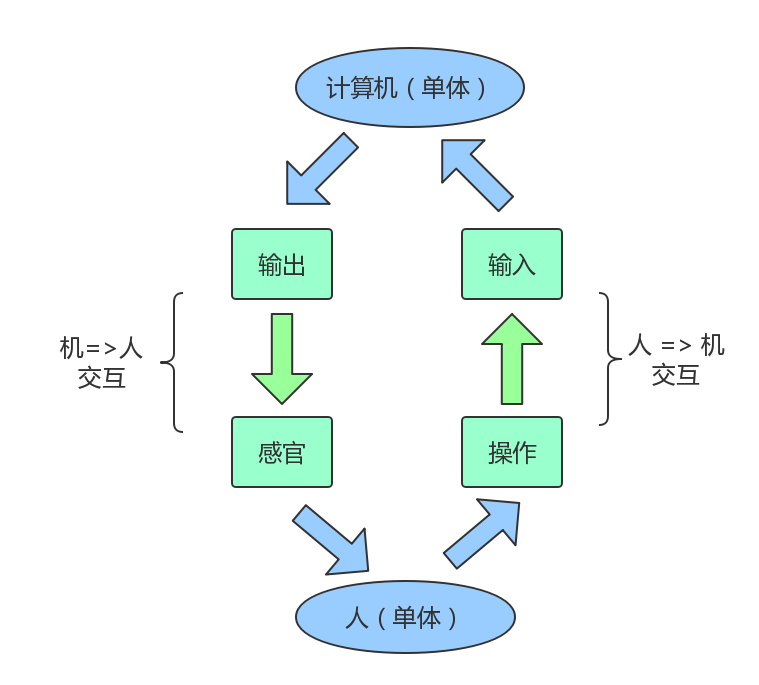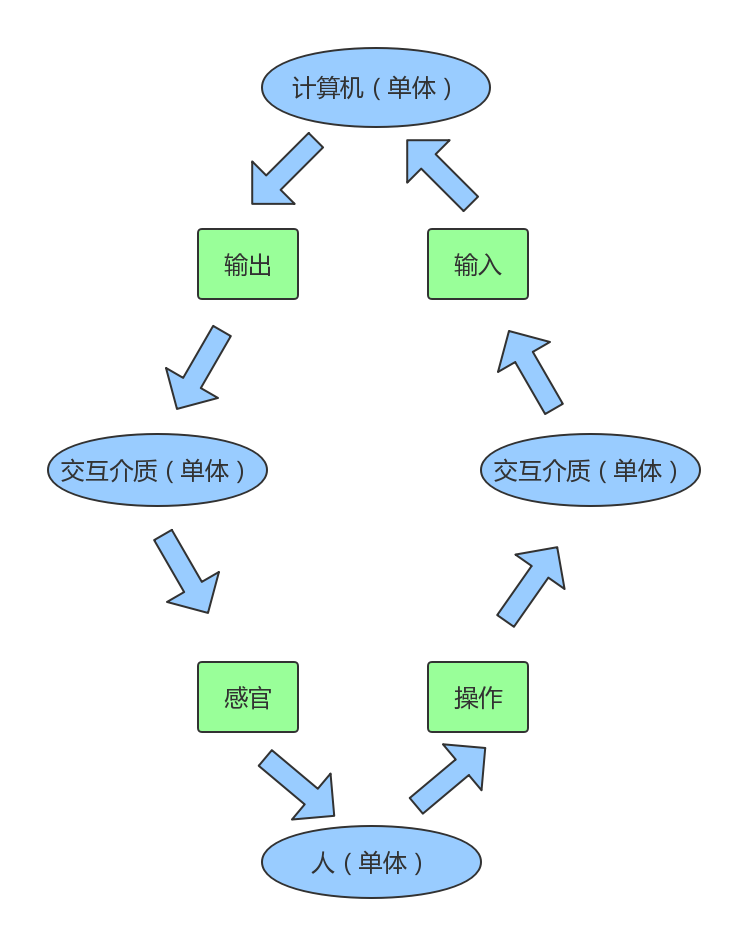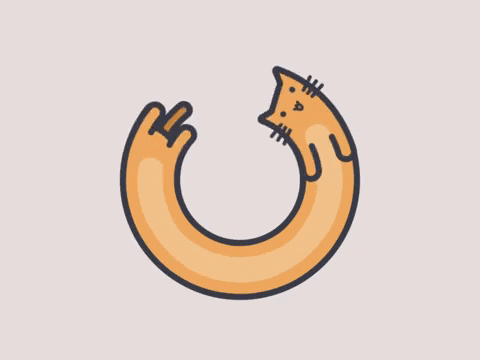# # 单体模型

## # 个体

``````function 人体(听觉, 视觉, 触觉, ...其他感觉) {
传递(听觉, 视觉, 触觉, ...其他感觉);
反馈 = 处理(听觉, 视觉, 触觉, ...其他感觉);
return 反馈;
}
``````

``````function 个体(输入) {
输出 = 处理(输入);
return 输出;
}
``````

## # 群体

### # 1. 宏观角度

``````群体 = 个体;
``````

### # 2. 微观角度

``````群体 = 个体 + ... + 个体 + 各个个体间的相互作用
``````
``````// 1. 假设个体间满足串行关系
function 群体(输入){
个体1输出 = 个体1处理(输入);
个体2输出 = 个体2处理(个体1输出);
...
个体n输出 = 个体n处理(个体n-1输出);
return 个体n输出;
}

// 2. 假设个体间满足并行关系
function 群体(输入){
// 拆分给各个个体的输入，分别处理
for(var i = 0; i < 个体.length; i++){
个体i输入 = 获取个体输入(输入, i);
个体i输出 = 个体i处理(个体i输入)
}
// 汇总输出
总输出 = 汇总([...各个个体的输出]);
return 总输出;
}
``````

``````// 单体模型
function 单体(输入) {
输出 = 处理(输入);
return 输出;
}
``````

# # 交互

## # 人机交互

• 人 => 机：通过操作对机器进行输入，例如从键盘输入内容、用鼠标点击某些元素等
• 机 => 人：机器拿到输入内容，根据程序进行计算，可通过显示器、声音设备进行输出``````function 人(输入) {
输出 = 处理(输入);
return 输出;
}

function 机(输入) {
输出 = 处理(输入);
return 输出;
}

// 1. 输入从机开始

// 2. 输入从人开始

``````

## # 多体交互

``````const 单体1输入 = 汇总(...其他n-1个单体的输出);
``````

``````function 交互介质(单体输出) {
单体输入 = 处理(单体输出);
return 单体输入;
}
``````## # 流

``````function 监听器(输入流, 单体) {
输入流.变更 = 输入流 => {
单体(输入流);
};
}
``````

``````单体n输出 = 单体n(...单体2(单体1(输入)));
``````

``````function 添加流处理(...n个单体){
// 注册流的链用方法
n个单体.forEach((单体, n) => {
流.单体n = function() {
this = 单体(this);
return this;
}
})
}

...

``````

``````输出流 = 输入流.单体1().单体2().[...].单体n();
``````

## # 光与电的世界

### # 光和电的单体

``````function 单体(输入) {
输入光 = 输入.光;
输出光 = 处理光(输入光);

输入电 = 输入.电;
输出电 = 处理电(输出电);

// 输出
return {
光: 输出光,
电: 输出电
};
}
``````

``````function 世界(输入) {
输入光 = 输入.光;
输出光 = 传播(输入光);

输入电 = 输入.电;
输出电 = 传播(输出电);

// 输出
return {
光: 输出光,
电: 输出电
};
}
``````

``````function 创建世界() {
const 输入 = {};
输入.光 = 添加光源();
输入.电 = 添加电源();
return 世界(输入);
}
``````

### # 光和电的传播机制

``````输出流 = 输入流.单体1().单体2().[...].单体n();
``````

1. 流的规则设定好，流开始流动；
2. 流的流动，表现为各单体对流的 输入 => 处理 => 输出；
3. 单体和世界、单体间通过流来进行交互，表现为 输入 => 处理 => 输出；
4. 其中每一段流作为研究对象，抽象成单体或者群体进行分析。

1. 对不同物质会产生不同的效果（单体的处理）。
2. 会在经过不同的物质之后，增强或是减弱（输入流和输出流）。

# # 结束语